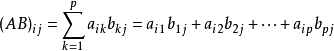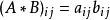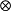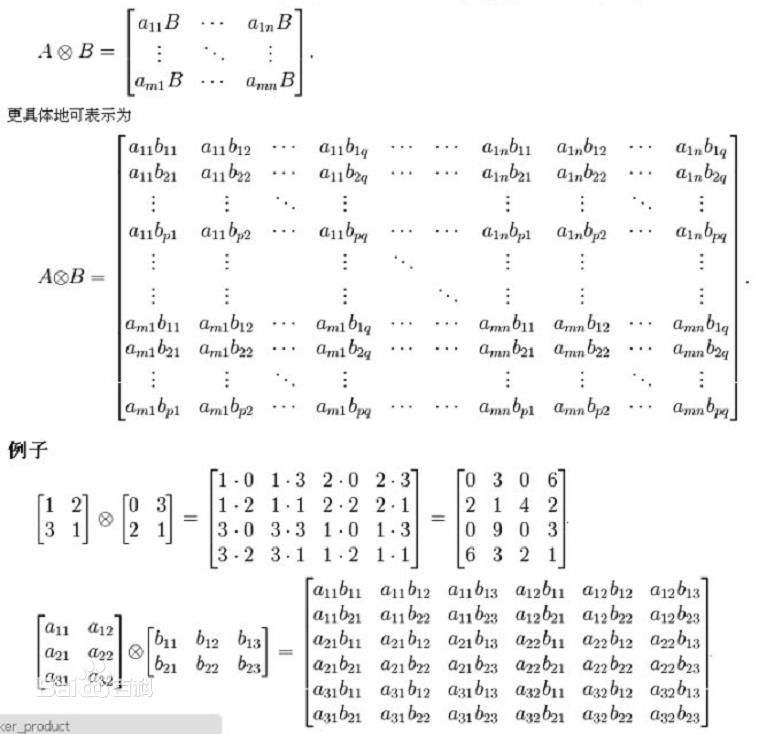• ## 张量(矩阵)乘积

万次阅读 2018-05-14 15:25:58
设A为m*p的矩阵，B为p*n 的矩阵，那么称m*n的矩阵C为矩阵AB的乘积， 记作 C = AB 其中矩阵C中的第 行第 列元素可以表示为： 这里写代码片 2 哈达马积 (hadamard product) m*n矩阵 A m*n矩阵 B 的Hadam.....
关于张量里面的积有太多形式了,这里终结一下,并用Python实现
1 矩阵乘积 (matmul product)
设A为m*p的矩阵，B为p*n  的矩阵，那么称m*n的矩阵C为矩阵A与B的乘积，
记作  C = AB
其中矩阵C中的第 行第  列元素可以表示为：这里写代码片

2 哈达马积 (hadamard product)
m*n矩阵 A 与m*n矩阵 B 的Hadamard积记为A*B.
其元素定义为两个矩阵对应元素的乘积:这里写代码片

3 克罗内克积 (Kronecker product)
Kronecker积是两个任意大小的矩阵间的运算，表示为,又称为直积或张量积.展开全文Python tensor
• 矩阵乘积状态Langevin方程描述了环境热接触系统轨迹。 它扩展了随时间变化变分原理，用于演化具有附加噪声和摩擦项的矩阵乘积状态。 安装 下载此存储库，并将其文件夹和所有子文件夹添加到MATLAB路径。 从...
• 点 - nd 数组乘法一个电话看起来像[ijkl] = 指数；... 该功能 Paolo de Vela “multiprod”非常相似。 目标是在 CPU 和 RAM 方面更经济地工作。 此外，调用语法是不同，但这显然是一个品味问题。matlab
• @TOC 一、Tensor-train分解 1. 定义 给定N阶张量，将其分解成N个二阶或三阶张量的缩并形式: 2. TT低秩近似 二、矩阵乘积态 1. 定义 2. 矩阵乘积量子纠缠 3. 矩阵乘积规范自由度正交形式
TT分解和矩阵乘积态一、Tensor-train分解（TT分解）1. 定义2. TT低秩近似二、矩阵乘积态（MPS）1. 定义2. 矩阵乘积态与量子纠缠3. 矩阵乘积态的规范自由度与正交形式
我是一只正在不断学习、希望早日成为小白的小小白，有什么错误欢迎大家批评指正，喜欢的请点个赞哦！
一、Tensor-train分解（TT分解）
1. 定义
给定N阶张量，将其分解成N个二阶或三阶张量的缩并形式:
$T_{S_{1} S_{2} \ldots S_{N}}=\sum_{a_{1} a_{2} \ldots a_{N-1}} A_{S_{1} a_{1}}^{(1)} A_{S_{2} a_{1} a_{2}}^{(2)} \ldots A_{S_{N-1} a_{N-2} a_{N-1}}^{(N-1)} A_{S_{N}}^{(N)}=A_{S_{1}:}^{(1)} A_{S_{2}: \cdots}^{(2)} \ldots A_{S_{N-1}::}^{(N-1)} A_{S_{N}:}^{(N) \mathrm{T}}$
其中，$\left\{ {{a}_{n}} \right\}$被称为几何或辅助指标，$\left\{ {{s}_{n}} \right\}$被称为开放或物理指标。
tensor-train直译过来就是张量火车，正如下图我们所看到的那样，一个大的张量被分解成了一个个小张量缩并的形式，就像我们平时所看到的一节节火车的车厢，所以不得不说前人还是很富有想象力的。为了方便，在后面的学习中我们会把Tensor-train分解简称为TT分解。TT分解可以通过N-1次奇异值分解实现。先把第一个指标看成一个指标，后面三个指标看成一个大指标，这样子就得到一个二阶张量（矩阵），将其进行奇异值分解，左边就是奇异值分解后得到的$U$，右边就是$\Lambda {{V}^{\dagger }}$。同理对右边的张量按照上述方法继续进行奇异值分解……例如四阶张量的TT分解，如下图：经过N-1次奇异值分解，一个N阶的张量就被分解成了N个小张量。但是严格的TT分解不能解决指数墙的问题，辅助指标会随着奇异值分解的次数呈指数增长。那么这个时候怎么解决这个问题呢？可以先思考下，答案后面揭晓。2. TT低秩近似
TT秩的定义
每一次进行奇异值分解所得到的非0奇异值的个数（辅助指标的维数），因为N阶张量要进行N-1次奇异值分解，所以一个N阶张量的TT秩包含N-1个数。TT低秩近似
令辅助指标有一个上限，辅助指标的上限 $\chi$ 称为截断维数或裁剪维数:
$\min _{\left\{\operatorname{dim}\left(a_{n}\right) \leq \chi\right\}} \mid T_{s_{1} s_{2} \ldots s_{N}}-\sum_{a_{1} a_{1} \ldots a_{N-1}} A_{s_{1} a_{1}}^{(1)} A_{s_{2}}^{(2)} a_{1} a_{2} \cdots A_{s_{N-1} a_{N-2} a_{N-1}}^{(N-1)} A_{S_{N} a_{N-1}}^{(N)}$
获得TT秩近似最简单的算法之一就是每次进行奇异值分解后将多于截断维数的奇异值和对应的奇异向量进行裁剪，将辅助指标的维数裁剪为 $\chi$ ，简而言之就是在进行TT分解时不断利用奇异值分解的低秩近似，是不是超级容易就解决了一个问题。
随机生成10×10×10×10的张量进行不同截断维数的TT低秩近似的误差（下图为 $\chi$ 取30到60）附上代码，没事可以自己去试试，感觉有点神奇呢！（看我这没见过世面的样子U•ェ•U）：
import numpy as np
import BasicFun as bf
import matplotlib.pyplot as plt

# 考虑(2, 2, 2, 2, 2, 2, 2, 2, 2, 2)的8阶张量，进行无裁剪TT分解，查看分解后辅助指标的维数
x = np.random.randn(2, 2, 2, 2, 2, 2, 2, 2, 2, 2)
tensors = bf.ttd(x)
# 读取辅助指标维数
a_dims = list()
for x in tensors[:-1]:
a_dims.append(x.shape[-1])
print('The ancillary dimensions of the TT form = ')
print(a_dims)

# 考虑(10, 10, 10, 10)的张量，计算不同截断维数下的误差
y = np.random.randn(10, 10, 10,10)
err = list()
z0 = np.linalg.norm(y)
for chi in range(30, 61,1):
tensors = bf.ttd(y, chi)
y1 = bf.tt_product(tensors)
err.append(np.linalg.norm(y - y1) / z0)
print('\nThe error with different dimension cut-off = ')
print(err)
plt.plot(np.arange(30, 61,1), err, marker='x')
plt.xlabel(r'dimension cut-off $\chi$')
plt.ylabel('relative error')
plt.show()

二、矩阵乘积态（MPS）
1. 定义
一般而言，矩阵乘积态（MPS）定义为系数矩阵满足TT形式的量子态:
$|\varphi\rangle=\sum_{s_{1} s_{2} \ldots s_{N}} \varphi_{s_{1} s_{2} \ldots s_{N}} \prod_{\otimes n=1}^{N}\left|s_{n}\right\rangle$
在不引起误解的情况下矩阵乘积态也可以直接理解为系数矩阵:
$\varphi_{s_{1} s_{2} \ldots s_{N}}=\sum_{a_{1} a_{1} \ldots a_{N-1}} A_{s_{1} a_{1}}^{(1)} A_{s_{2} a_{1} a_{2}}^{(2)} \ldots A_{s_{N-1} a_{N-2} a_{N-1}}^{(N-1)} A_{S_{N} a_{N-1}}^{(N)}=A_{s_{1}:}^{(1)} A_{S_{2}: \cdots}^{(2)} \ldots A_{S_{N-1}:}^{(N-1)} A_{S_{N}:}^{(N) \mathrm{T}}$
矩阵乘积态的两种边界条件分别为开发边界条件（OBC）和周期边界条件（PBC），如下图：在TT分解中，量子态参数个数随N指数增加，满足:
$\text{ }\!\!\#\!\!\text{ }\left( \left| \varphi \right\rangle \right)\sim O\left( {{d}^{N}} \right)$
在MPS中，给定辅助指标截断维数为 ，则MPS包含参数的个数随N线性增加，满足：
$\text{ }\!\!\#\!\!\text{ }\left( MPS \right)\sim O\left( Nd{{\chi }^{2}} \right)$
对于MPS分解，我们并不需要知道指数复杂的量子系数是什么，也不需要进行TT分解，而是直接假设基态具备MPS的形式，直接处理MPS中的局部张量，从而绕开指数墙的问题，但是这样子会引入误差。并且，在求基态时我们是在并不知道基态是什么的情况下直接假设基态是具有给定截断维数的MPS态，因此我们并不能确定这样的MPS态可以有效的描述基态。我们可以从奇异谱入手定义刻画MPS的有效性，而MPS态对应的奇异谱刚好就是量子态的量子纠缠谱，由此引入量子纠缠。有没有很懵，don’t worry，继续往下看！2. 矩阵乘积态与量子纠缠
斯密特分解与纠缠谱：给定N自旋的量子态
$\left| \varphi \right\rangle =\sum\nolimits_{{{s}_{1}}{{s}_{2}}\cdots {{s}_{N}}}{{{\varphi }_{{{s}_{1}}{{s}_{2}}\cdots {{s}_{N}}}}\prod\limits_{\otimes n=1}^{N}{\left| {{s}_{n}} \right\rangle }}$
将自旋分成两部分:
$\left\{ {{s}_{n}} \right\}=\left( {{s}_{1}},{{s}_{2}},\cdots ,{{s}_{K}} \right)\cup ({{s}_{K+1}},\cdots ,{{s}_{N}})$
两部分不能为空，将前面一部分看成一个指标，后面一部分看成一个指标，这样就得到一个二阶张量（矩阵），对矩阵的系数进行奇异值分解：
${{\varphi }_{{{s}_{1}}{{s}_{2}}\cdots {{s}_{N}}}}=\sum\limits_{a=0}^{D-1}{{{U}_{{{s}_{1}}{{s}_{2}}\cdots {{s}_{K}},a}}{{\Lambda }_{a}}}V_{{{s}_{K+1}}\cdots {{s}_{N}},a}^{*}$
对系数进行奇异值分解对应于对量子态做分解：
$\left| \varphi \right\rangle =\sum\limits_{a=0}^{D-1}{{{\Lambda }_{a}}\left| {{U}^{a}} \right\rangle \left| {{V}^{a}} \right\rangle }$
$\left| {{U}^{a}} \right\rangle \left| {{V}^{a}} \right\rangle$之间进行直积，${{\Lambda }_{a}}$可看成权重系数。该分解被称为量子态的斯密特分解，量子态的斯密特分解对应于系数矩阵的奇异值分解，$\Lambda$称为量子态的纠缠谱。其中$\left| {{U}^{a}} \right\rangle \left| {{V}^{a}} \right\rangle$为D个量子态，满足：
$\left| {{U}^{a}} \right\rangle =\sum\limits_{{{s}_{1}}{{s}_{2}}\cdots {{s}_{K}}}^{D-1}{{{U}_{{{s}_{1}}{{s}_{2}}\cdots {{s}_{K}},a}}\prod\limits_{\otimes n=1}^{K}{\left| {{s}_{n}} \right\rangle }}$
$\left| {{V}^{a}} \right\rangle =\sum\limits_{{{s}_{1}}{{s}_{2}}\cdots {{s}_{K}}}^{D-1}{{{V}^{*}}_{{{s}_{K+1}}\cdots {{s}_{N}},a}\prod\limits_{\otimes n=1}^{K}{\left| {{s}_{n}} \right\rangle }}$
由于
$\left\langle \varphi \right|\left. \varphi \right\rangle \text{=1}，\left| \varphi \right\rangle \text{=}\sum\limits_{a=0}^{D-1}{{{\Lambda }_{a}}\left| {{U}^{a}} \right\rangle \left| {{V}^{a}} \right\rangle }$
所以
$\left\langle \varphi \right|\left. \varphi \right\rangle \text{=}\sum\limits_{a=0}^{D-1}{{{\Lambda }_{a}}^{\text{2}}\left\langle {{V}^{a}} \right|\left\langle {{U}^{a}} \right.\left| {{U}^{a}} \right\rangle \left| {{V}^{a}} \right\rangle }\text{=}\sum\limits_{a=0}^{D-1}{{{\Lambda }_{a}}^{\text{2}}\text{=1}}$
定义$\left| \varphi \right\rangle$被投影到$\left| {{U}^{a}} \right\rangle \left| {{V}^{a}} \right\rangle$态的概率满足${{P}_{a}}=\Lambda _{a}^{2}$（量子力学的基本假设），显然概率满足归一化条件
$\sum\nolimits_{a}{{{P}_{a}}=1}$
根据概率论香农熵的定义：
${{E}^{S}}=-{{P}_{a}}\sum\nolimits_{a}{\ln {{P}_{a}}}$
根据该公式定义量子态的纠缠熵：
$S=-\sum\limits_{a=0}^{D-1}{\Lambda _{a}^{2}}\ln \Lambda _{a}^{2}$
因为香农熵是刻画信息量的大小，类推到量子态，那么量子态的纠缠熵就是刻画量子信息量的大小。把量子态二分为A体系和B体系，A、B体系在进行给定基底下的概率测量得到的信息熵就是这两个体系在该二分下的纠缠熵。
奇异值分解中
${{\varphi }_{{{s}_{1}}{{s}_{2}}\cdots {{s}_{N}}}}=\sum\limits_{a=0}^{D-1}{{{U}_{{{s}_{1}}{{s}_{2}}\cdots {{s}_{K}},a}}{{\Lambda }_{a}}}V_{{{s}_{K+1}}\cdots {{s}_{N}},a}^{*}$
$UV$满足正交性：
$U^{\dagger} U=\sum_{s_{1}\ldots S_{K}} U_{s_{1} \ldots S_{K}, \alpha}^{*} U_{S_{1} \ldots S_{K}, \alpha^{\prime}}=I$
$V^{\dagger} V=\sum_{s_{1}\ldots S_{K}} V_{s_{1} \ldots S_{K}, \alpha}^{*} V_{S_{1} \ldots S_{K} \alpha^{\prime}}=I$
由上述性质可计算开放边界MPS的纠缠，设MPS态满足：
$\varphi_{s_{1} s_{2} \ldots s_{N}}=\sum_{a_{1} a_{1} \ldots a_{N-1}} A_{s_{1} a_{1}}^{(1)} \ldots A_{s_{K} a_{K-1} a_{K}}^{(K)} \Lambda_{a_{K}}^{(K)} A_{s_{K+1} a_{K} a_{K+1}}^{(K+1)} \ldots A_{S_{N} a_{N-1}}^{(N)}$
当如下左/右正交条件满足时，${{\Lambda }^{K}}$为MPS给出的前K个自旋与其余自旋之间的纠缠：
a. $\sum_{s_{1}} A_{s_{1} a_{1}}^{(1)} A_{s_{1} a_{1}}^{(1) *}=I_{a_{1} a_{1}}$
b. $\sum_{s_{n} a_{n-1}} A_{s_{n} a_{n-1} a_{n}}^{(n)} A_{s_{n} a_{n-1} a_{n}}^{(n) *}=I_{a_{n} a_{n}} \quad(1
$\mathrm{c.} \sum_{s_{N}} A_{s_{N} a_{N-1}}^{(N)} A_{S_{N} a_{N-1}}^{(N) *}=I_{a_{N-1} a_{N-1}}$
$\operatorname{d.} \sum_{s_{n} a_{n+1}} A_{s_{n} a_{n-1} a_{n}}^{(n)} A_{s_{n} a_{n-1} a_{n}}^{(n) *}=I_{a_{n-1} a_{n-1}}(K
e. $\Lambda_{0}^{(K)} \geq \Lambda_{1}^{(K)} \geq \cdots \geq 0$
前面K个tensor收缩构成SVD分解中的U，后面的tensor收缩构成SVD分解中的V，称为MPS的中心正交形式，也称为SVD形式。例如长度为4的MPS，箭头表示正交条件的方向：MPS的中心正交形式在后面的学习中会经常涉及，且与SVD分解紧密联系在一起，所以这个要好好进行理解消化(　o=^•ェ•)o！
3. 矩阵乘积态的规范自由度与正交形式
计算MPS的斯密特分解可将MPS变成SVD形式，但是其所表示的量子态不能改变。改变MPS中的tensor但不改变其所表示的量子态，被称为规范变换也就是物理量不改变、数学形式改变的变化。进一步定义MPS的规范自由度：对于同一个量子态，可由多组由不同张量组成的MPS态来表示其系数。
已知MPS满足：
$\varphi_{S_{1} S_{2} \ldots S_{N}}=A_{S_{1}:}^{(1)} \ldots A_{S_{n}:}^{(n)} A_{S_{n+1}: \cdots}^{(n+1)} \ldots A_{S_{N}:}^{(N) \mathrm{T}}$
引入任意可逆矩阵$U$及其逆矩阵${{U}^{-1}}$，定义:
$B_{{{s}_{n}}}^{(n)}=A_{{{s}_{n}}::}^{(n)}U,B_{{{s}_{n}}}^{(n+1)}={{U}^{-1}}A_{{{s}_{n}}::}^{(n+1)}$
得到同一个量子态的两种MPS表示:
$\varphi_{s_{1} s_{2} \ldots s_{N}}=A_{s_{1}}^{(1)} \ldots A_{s_{n}::}^{(n)} A_{s_{n+1}: \cdots}^{(n+1)} \ldots A_{s_{N}:}^{(N) \mathrm{T}}=A_{s_{1}:}^{(1)} \ldots B_{s_{n}:}^{(n)} B_{s_{n+1} !}^{(n+1)} \ldots A_{S_{N}:}^{(N) \mathrm{T}}$
公式总是没有图来得直观，下面我们用下图来进行进一步理解。MPS规范变换可以在任何一个或多个辅助指标上插入一个单位阵，也就是任何可逆矩阵及其逆矩阵的乘积。我们都知道任何矩阵乘单位阵都等于自己本身，所以在任何一个或多个辅助指标上插入一个单位阵都不会改变量子态。将变换矩阵作用到各张量{${{A}^{(n)}}$}上，就能得到新张量{${{B}^{(n)}}$}在这种情况下，我们插入不同维数的单位矩阵就会得到不同的新张量{${{B}^{(n)}}$}，这个时候，我们可以通过引入新的约束条件，固定MPS的规范自由度，使得给定量子态具备唯一的MPS表示。
例如MPS的中心正交形式，如下图所示。当张量{${{A}^{(n)}}$}（n<k）满足左正交条件，{${{A}^{(n)}}$}（n>k）满足右正交条件，中间的tensor满足归一条件，此时MPS被称为具有$K$-中心正交形式。可通过多次的SVD分解进行规范变换从$K$-中心正交形式变换成K‘-中心正交形式（$K\ne K'$），这个过程值得细细思考品味。给定任意一个矩阵乘积态，求量子纠缠谱（${{\Lambda }^{(K)}}$）：
（1）	把该矩阵乘积态变成K-中心正交形式；
（2）	对K-中心正交形式的中心tensor做奇异值分解。
基于K-中心正交形式，可对MPS辅助指标维数进行最优裁剪，裁剪方法为：
（1）	进行中心正交化，将正交中心放置于第K个张量；
（2）	对中心张量进行奇异值分解
$A_{{{s}_{K}}{{a}_{K-1}}{{a}_{K}}}^{(K)}=\sum\nolimits_{\beta =0}^{\chi -1}{{{U}_{{{s}_{K}}{{a}_{K-1}}\beta }}\Lambda _{\beta }^{(K)}{{V}_{{{a}_{K}}\beta }}}$
仅保留前$\chi$个奇异值及对应的奇异值向量，其中$\chi$为截断维数；
（3）	将第K个张量更新为$U\to {{A}^{(K)}}$;
（4）	将第（K+1）个张量更新为
$\sum\nolimits_{{{a}_{K}}}{\Lambda _{\beta }^{(K)}{{V}_{{{a}_{K}}\beta }}}A_{{{s}_{K+1}}{{a}_{K}}{{a}_{K+1}}}^{(K+1)}\to A_{{{s}_{K+1}}\beta {{a}_{K+1}}}^{(K+1)}$
通过不断移动正交中心的位置来实现MPS辅助指标的最优裁剪。
MPS的正则形式：给定量子态
$\left| \varphi \right\rangle =\sum\nolimits_{{{s}_{1}}{{s}_{2}}\cdots {{s}_{N}}}{{{\varphi }_{{{s}_{1}}{{s}_{2}}\cdots {{s}_{N}}}}\prod\limits_{\otimes n=1}^{N}{\left| {{s}_{n}} \right\rangle }}$
其系数满足：
$\varphi_{S_{1} S_{2} \ldots S_{N}}=A_{S_{1}:}^{(1)} \Lambda^{(1)} A_{S_{2}: \Lambda}^{(2)} \Lambda^{(2)} \ldots \Lambda^{(N-2)} A_{S_{N-1}: \Lambda}^{(N-1)} \Lambda^{(N-1)} A_{S_{N}:}^{(N) \mathrm{T}}$
${{\Lambda }^{(n)}}$是当前位置下进行二分得到的纠缠谱，满足:
a. $\sum_{s_{1}} A_{s_{1} a_{1}}^{(1)} A_{s_{1} a_{1}}^{(1) *}=I_{a_{1} a_{1}}$
b. $\sum_{s_{n} a_{n-1}} \Lambda_{a_{n-1} a_{n-1}}^{(n-1)} A_{s_{n} a_{n-1} a_{n}}^{(n)} \Lambda_{a_{n-1} a_{n-1}}^{(n-1)} A_{s_{n} a_{n-1} a_{n}}^{(n) *}=I_{a_{n} a_{n}}$
c. $\sum_{s_{n} a_{n}} A_{s_{n} a_{n-1} a_{n}}^{(n)} \Lambda_{a_{n} a_{n}}^{(n)} A_{s_{n} a_{n-1} a_{n}}^{(n) *} \Lambda_{a_{n} a_{n}}^{(n)}=I_{a_{n-1} a_{n-1}} \quad(1
d. $\sum_{S_{N}} A_{S_{N}}^{(N)} a_{N-1} A_{S_{N} a^{\prime} N-1}^{(N) *}=I_{a_{N-1} a_{P-1}}$有没有觉很很神奇，神奇的事情还在后面，我们下期见！
我是一只正在不断学习、希望早日成为小白的小小白，有什么错误欢迎大家批评指正，喜欢的请点个赞哦！展开全文python 量子力学 机器学习
• 当前，scikit-tensor支持基本的张量操作，例如折叠/展开，张量矩阵张量向量乘积以及以下张量分解： 规范/ Parafac分解 塔克分解 RESCAL 迪迪康 印度INDSCAL 而且，所有操作都支持密集和张量。 笔记 这是仅Python...
• #所以需要注意的是 张量 不是 节点操作...#张量在 定义完成之后是不会进行操作的，想要进行操作就必须使用 节点操作 也就是OP来运行，才能计算出ab矩阵的乘积   #OP其实是在描述 张量 中的运算关系 可以参...
#所以需要注意的是 张量 不是 节点操作(OP)

#tensor1 = tf.matmul(a,b,name='exampleop')

#上面这个  定义的只是一个张量，是在一个静态的图(graph)中的

#张量在 定义完成之后是不会进行操作的，想要进行操作就必须使用  节点操作  也就是OP来运行，才能计算出ab矩阵的乘积

#OP其实是在描述  张量  中的运算关系

可以参照下面的这个例子，来理解tensorflow中  张量  与  节点操作  之间的关系

import tensorflow as tf

tf.reset_default_graph()

a = tf.constant([[1.0,2.0]])
b = tf.constant([[1.0],[3.0]])

tensor1 = tf.matmul(a,b,name='exampleop')
print(tensor1.name,tensor1)

g3 = tf.get_default_graph()

#注意 tensor的名字是定义的 name 再加上  :0，也就是   name:0
#把找到的tensor复制给这个变量，也就是说test和tensor1是一样的
test = g3.get_tensor_by_name('exampleop:0')
print(test)

print(tensor1.op.name)

#获取节点操作 OP
testop = g3.get_operation_by_name("exampleop")
print("a:",a.name)
print("b:",b.name)
print(testop)

with tf.Session() as sess:
sess.run(tf.global_variables_initializer())

test = sess.run(test)
print(test)

test = tf.get_default_graph().get_tensor_by_name("exampleop:0")
print(test)



展开全文节点操作 tensorflow
• ITensor库还包括可组合和可扩展算法，用于优化和转换张量网络，例如矩阵乘积状态和矩阵乘积运算符，例如DMRG算法。 西蒙斯基金会下属熨斗研究所（Flatiron Institute）支持ITensor开发。 安装 ITensors...
• 在前面两个工作的基础上,利用设计的连分式算法逼近张量指数函数,其特点在于,该算法可以编程实现递推计算,而且在计算过程中不必计算张量的乘积,也不必计算张量的逆.给出的两个张量指数函数的数值实验表明,将连分式...
•   在上一次学习中，我们从TT分解借助新的张量表示方法过渡到了矩阵乘积态，可以说矩阵乘积态（MPS）另一个别称为TT分解，现在，让我们再一次看看以下两个概念。 截断维数χ\chiχTT秩：截断维数实际上就是...
  在上一次的学习中，我们从TT分解借助新的张量表示方法过渡到了矩阵乘积态，可以说矩阵乘积态（MPS）的另一个别称为TT分解，现在，让我们再一次看看以下两个概念。

截断维数$\chi$与TT秩：截断维数实际上就是TT分解（或MPS）中辅助指标能取的数的个数（最多的），然后借此引入了TT秩，它是一个n维数列（向量），里面每个元素就是每个辅助指标所存在的能取的最小的个数截断维数 ，TT秩是在不作任何近似时引入的概念，但如果不作任何近似，随着张量阶数增加，截断维数会指数增加，这样下来，我们遇到了“指数墙”问题，然后我们又极小化辅助指标，这就是最优化TT低秩近似，但这并未解决“指数墙”问题。
矩阵乘积态（MPS）：给定的是一个量子态，将其表示为多个基态（自旋）的叠加，每个基态前面的系数满足TT形式，然后我们就可以直接假设一个量子（多体）态可以表示成一个MPS态，然后我们不关心原来的那个完整的量子态的具体情况，而是直接去处理MPS态，如此就绕过了“指数墙”，但也由此引入了误差，我们怎么去衡量误差，或者说这个MPS态的有效性？那就得考虑奇异谱了，接着又引入了斯密特分解，我们可以借此得到纠缠谱。（我们还可以借助纠缠谱和香农熵的定义来定义并计算纠缠熵，纠缠熵刻画信息量的大小，刻画误差的大小，也可以看这个MPS态的有效性如何）

所以现在，我们想要计算MPS态的斯密特分解来得到纠缠谱进而计算纠缠熵来衡量这个绕过了指数墙的MPS态的有效性的大小。
一.纠缠熵
纠缠熵
对于一条信息，我们很难知道这条信息带给了我们什么，或者说这条信息内在的信息量多大，然后又发现一条信息的信息量大小和它的不确定性有直接的关系，由此引入了香农熵，借助香农熵，我们定义纠缠熵：我们暂时不用去仔细关注这两个概念，只要知道纠缠谱和纠缠熵密切相关，纠缠熵又与本次学习所要达到的目的——衡量误差密切相关，接下来才是正餐。
量子纠缠
在MPS态中，我们考虑每个基态都是自旋，这也意味着系数（概率幅）满足归一化条件，这和香农熵以及纠缠熵是相匹配的，又因为MPS可以借助SVD分解，里面的矩阵满足正交性条件，于是给定一个MPS态，其系数：当满足以下正交条件：$\Lambda$k为MPS给出的前K个自旋与其余自旋之间之间的纠缠。
二.矩阵乘积态的规范自由度和正交形式
矩阵乘积态的规范自由度
为了计算MPS态的斯密特分解，我们将满足左正交条件的矩阵收缩为SVD中的左奇异矩阵，满足右正交条件的矩阵收缩为SVD中的右奇异矩阵，即将MPS变换成SVD形式，这样的操作不会改变原量子态，故称为规范变换；同一个量子态可由多组由不同的张量组成的MPS态来表示量子态的系数，这称为规范自由度，这其实就像许多人鼓励其他人改变内在而不必执着于外在。
开放边界条件下MPS态的规范变化以下图为例：这张图很直观，红方块和蓝蓝方块互为可逆矩阵，它们的矩阵乘积为单位阵，如果我们不规定约束条件，不固定MPS态的规范自由度，就会使得MPS态的表示不止一种，而一般的约束条件就是前面的左右正交条件。.
正交形式

中心正交形式
当张量{𝐴(𝑛) }（ 𝑛<𝐾 ）满足左正交条件，{𝐴(𝑛) }（ 𝑛>𝐾 ）满足右正交条件时，MPS被称为具有𝐾-中心正交形式。红色箭头代表正交条件的方向。
正则形式
给定量子态：其系数满足：其中 $\Lambda$n为正定对角阵，以长度为4的MPS态为例，它的图形表示如下：这里实际上进行了3次奇异值分解，按从左往右SVD来看， $\Lambda$1就是第一个对角阵，相当于第一个二分纠缠谱，对比中心正交形式的并未直接显示二分纠缠谱（而是借助中心张量求得中心张量的奇异值谱，这个奇异值谱就是二分纠缠谱），正则形式可能会更方便。

接下来主要讨论中心正交形式以及基于此的截断维数的最优裁剪（由矩阵SVD低秩近似的最优性保证，是否有种似曾相识的感觉）：

正交中心的移动：通过多次的SVD或QR分解进行规范变换，到目前为止，我们讨论的一直都是SVD分解。
利用中心正交形式计算MPS的纠缠谱：借助中心张量求得中心张量的奇异值谱，这个奇异值谱就是二分纠缠谱。
基于中心正交形式的截断维数的最优裁剪：这种最优裁剪其实主要就是在SVD分解过程中奇异值矩阵只保留前$\chi$个奇异值和对应的奇异向量，$\chi$就是截断维数，其基本步骤是首先确定要裁剪的第K个辅助指标，然后将MPS态中心正交化，将正交中心移动至第k个张量，再对这个张量进行奇异值分解，最后更新第K和K+1个张量。

三.提出问题
现在让我们考虑以下几个问题：

斯密特分解时的系数矩阵中的元素是什么？怎么排列的？
有关MPS态绕过“指数墙”的问题？
截断维数$\chi$是怎么确定的？
一个高阶张量矩阵化后的元素怎么排列的？

上面的这些问题，有些是比较模糊的，可能涉及更细节的东西，有些现在我也只知道部分答案，在以后的每次博客，我会提出一些关于此次博客的问题，并尽量在下次或再往后的博客中解答，也会指定下次博客的方向，我相信这会是一个好的开始，下次学习的主角是TEBD算法。
参考资料链接：https://www.bilibili.com/video/BV17z411i7yM/


展开全文• 《多重线性代数与矩阵:王伯英文集》收入了《关于求逆矩阵方法一个注记》《一种降低条件数迭代格式》《一类矩阵特征值最小距离界限》《张量空间对称化算子指标》《正半定Hermitian矩阵乘积的一些特征值不等式...
• 与矩阵分解一样，我们希望通过张量分解去提取原数据中所隐藏信息或主要成分。当前主流的张量分解方法有CP分解，Tucker分解，t-SVD分解等，更新方法大多是在他们基础上做进一步改进或引用。因此，张量分解也...大数据 数据挖掘 深度学习 算法
• 最小化编译时间操作，例如图形优化，贪婪的矩阵乘积和近似符号操作，以将BLAS或非BLAS类型表达式计算复杂度降低几个数量级 显式和可配置SIMD向量化，支持所有数值数据类型float32 ， float64 ， complex float...
• 本文是对论文Tensor Decompositions and Applications进行了翻译、整理、筛选和...Tucker分解可以看作是主成分分析(PCA)的一种高阶版本，其将张量分解为一个核张量与每一维度上对应矩阵的乘积。具体来说，以三阶张量...深度学习 Tucker
• 矩阵乘法多维泛化 此函数根据爱因斯坦求和约定（加上附加项）计算广义多维矩阵乘积。 这意味着给定第二个输入： X = [ A x B x C x E .... ] Y = [ D x F x G x H .... ] 我们将结果 Z 定义为（在 ESC 中） Z_{c,e...matlab
• 在讲解Tucker分解之前，我们先看一下Tucker...它将一个张量分解成一个核张量与每一维矩阵的乘积，具体如下： 这里A∈RI×P,B∈RJ×Q,A∈RK×RA∈RI×P,B∈RJ×Q,A∈RK×RA\in R^{I\times P},B\in R^{J\times Q...Tucker分解
• 2.张量的定义运算 2.1 张量(Tensor) 2.2 纤维(Fibre) 2.3 切片(Slice) 2.4 内积(Inner product) 2.5 矩阵展开(Unfolding-Matricization) 2.6 外积(Outer Product) 2.7 Kronecker乘积(Kron...
• B 1及其无限梯度对称乘积符号（×B 1）∞情况分别对应于通常高自旋理论及其多粒子扩展。 推测多粒子B 2高自旋理论弦理论相关。 预期p> 2B p-高自旋模型是对rank-p边界张量sigma模型对偶。 具有p≥2B p...
• 基本矩阵操作np.add() 求和np.subtract()相减np.multiply() 乘积np.divide()相除矩阵的点积 np.dot()矩阵和向量之间的运算三维张量和矩阵之间的点积三维张量和向量运算六、ndarray的排序（数据结构...算法 数据结构 数据挖掘 python 快速排序
• ﻿﻿ 矩阵AKronnecker积也叫张量积，或矩阵A与矩阵B直积。 如果A是一个 m x n 矩阵，而B是一个 p x q 矩阵，克罗内克积则是一个 mp x nq 矩阵
• 该函数提供了一种使用爱因斯坦求和约定来计算多线性表达式（即乘积和）方法。 Parameters: 等式（string） - 该等式根据操作数和结果每个维度相关联小写字母（索引）给出。左侧列出了操作数尺寸，以逗号...
• 点积：两个维度相同向量相乘，可以看成A列和B行相乘，与矩阵相乘不同，点积满足交换律，点积结果是标量 元素对应乘积（element-wise product）是对应元素相乘。 单位矩阵：主对角线全是1，其他位置都是0。 ...
• 哈达马积（Hadamard product） 两个矩阵对应元素的乘积 * 卷积操作一般就是这个符号 克罗内克积（Kronecker Product）克罗内克积也被称为直积或张量积 x外面一个圈 https://baike.baidu.com/item/%E7%9F%A9%E9%98%
• 由于之前我学习过高等代数和解析几何，所有在此只列举不熟悉或容易忘记的...元素对应乘积（Hadamard乘积）：两个矩阵中对应元素的乘积矩阵乘积满足结合律，但是不满足交换律。 标量可以看作只有一个元素的矩阵，标深度学习 线性代数 机器学习
• 使用新的矩阵乘积，即半张量积，逻辑函数以其矩阵形式表示。 从其矩阵表达式中，可以获取一个标准来测试逻辑函数是否为渠化函数。 然后获得一个公式来计算渠化函数数量。 此外，提出了一种生成泛化函数算法。 ...
• 1.pytorch广播机制numpy类似，当两个tensor逐元素相乘时（两个...在pytorch中，*表示逐元素相乘，而@是矩阵乘积，但是对于高维tensor，@不再适用，尤其是对于size大小还不同两个张量，pytorch还有一个函数：mu机器学习
• 我们考虑了经典的瞬时张量SO（4）中三个具有相对旋转矩阵的D形大脑相关联的开弦构造的贡献，这对应于三个非阿贝尔扭曲场相关器的经典部分的计算。 我们将经典解写为两个超几何函数的乘积之和。 以前的所有三个...
• 一、标量、向量、矩阵张量 1、标量：一个标量就是一个单独数。 2、向量：一个向量就是一列数，且是有序排列。 （注：如果每个元素都属于R,并且向量有n个元素，那么该向量属于实数集Rn次笛卡尔乘积构成...
• 在此框架下，最重要O $$\ mathcal {O}$$（GFα）辐射校正由单个张量Tμν描述，该张量涉及带电弱电流和电磁电流之间时间乘积，所有剩余 在手性摄动理论中，每个片段都是可以按顺序计算。 我们进一步...
• 1标量、向量、矩阵张量标量（scalar）向量(vector)矩阵(matrix)张量(tensor)转置矩阵加法数乘矩阵矩阵乘法元素对应乘积单位矩阵矩阵用代码来实现标量、向量、矩阵线性相关向量线性组合、线性表示向量组线性...
• 最近写文章时，有些公式尤其涉及到...1.矩阵的对应乘积/Hadamard乘积 A⊙B 表示AB中的元素按位置对应相乘 2.标量 / 向量 / 矩阵 / 张量的区别 其中张量是表示多维度的数据网格 3.矩阵奇异 非满秩 4.L1 L2...